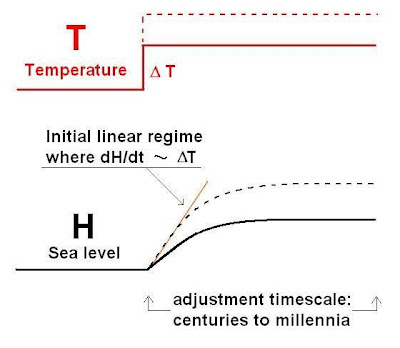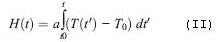## Critique of "A Semi-Empirical Approach to Projecting Future Sea-Level Rise" by Rahmstorf

August 12, 2007

A recent article in Science by Stefan Rahmstorf (2007) predicted extreme sea level rise during the 21st century. Rahmstorf’s predictions went as high as 140 cm (55 inches), far beyond even the high edge of the uncertainty of the IPCC’s unlikely A1Fl scenario (see here, page 820). This high estimate by the IPCC was 59cm (23 inches), with other other scenarios yielding considerably lower estimates. Following is a critique of Rahmstorf’s method and conclusions.

This post has a quick summary of Rahmstorf’s approach to to projecting sea-level rise for this century. Following that summary is a quick list of problems that I have identified in his paper, each with a link to subsequent posts with more detailed information.

Rahmstorf’s Simple Model

Rahmstorf’s simple model of sea level rise consists of a system in equilibrium, where the sea level and the temperature start out as constants. Then an instantaneous step occurs in the temperature, causing the sea level to rise. Eventually the sea level will rise to a new equilibrium, as shown below.It is very important to note that the time required to arrive at the new equilibrium is, according to Rahmstorf, “to be on the order of millennia.” This long time scale provides the other important point of this simple model. That is, over a short enough time scale the rate of sea level rise can be considered a constant (as illustrated in the above graph during the time where dH/dT is proportional to delta T). Rahmstorf posits that “this linear approximation may be valid for a few centuries.”

Therefore, in this model, a temperature jump in the 1920s, for example, would result in a sea level rising at a constant rate for several hundred years, even without any subsequent temperature increases. Of course, subsequent temperature rises would each result in a greater sea level rise rate, but there would never be any drop in the rise rate for several hundred years, assuming no significant drops in the temperature. The following section puts this model on a mathematical footing.

Rahmstorf’s Mathematical Strategy

1) Assume that the rate of sea level rise rate at any given time is proportional to the deviation form some global equilibrium temperature at that time. He expresses this in the following formula…where H is the sea level, dH/dt is the sea level rise rate, T is the temperature, To is the the equilibrium temperature, and a is the constant of proportionality.

2) To and a can be derived by simply plotting dH/dt vs T and fitting to a line.

3) Once To and a have been determined, then the sea level for any given time, H(t), can be calculated by integrating equation (I), above, with respect to time…4) By applying various temperature rise scenarios for the 21st century to equation (II), Rahmstorf predicts the sea level for the hear 2100 (H(2100)).

Problems with this model

1) Sea level rise rate vs. temperature is displayed in a way that erroneously implies that it is well fit to a line, as expressed in equation I, above. More…

2) The assumption that the time required to arrive at the new equilibrium is “on the order or millennia” is not borne out by the data. More…

3)Rahmstorf extrapolates out more than five times the measured temperature domain. More…